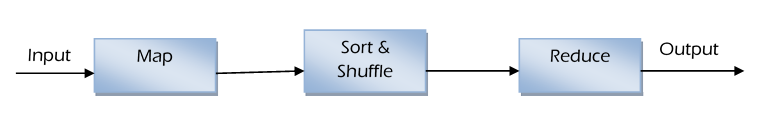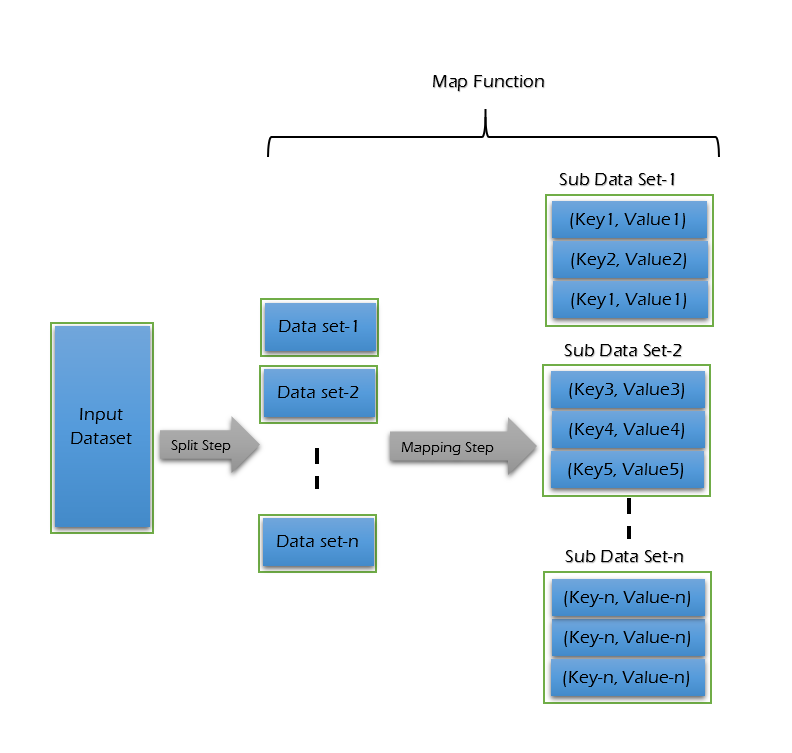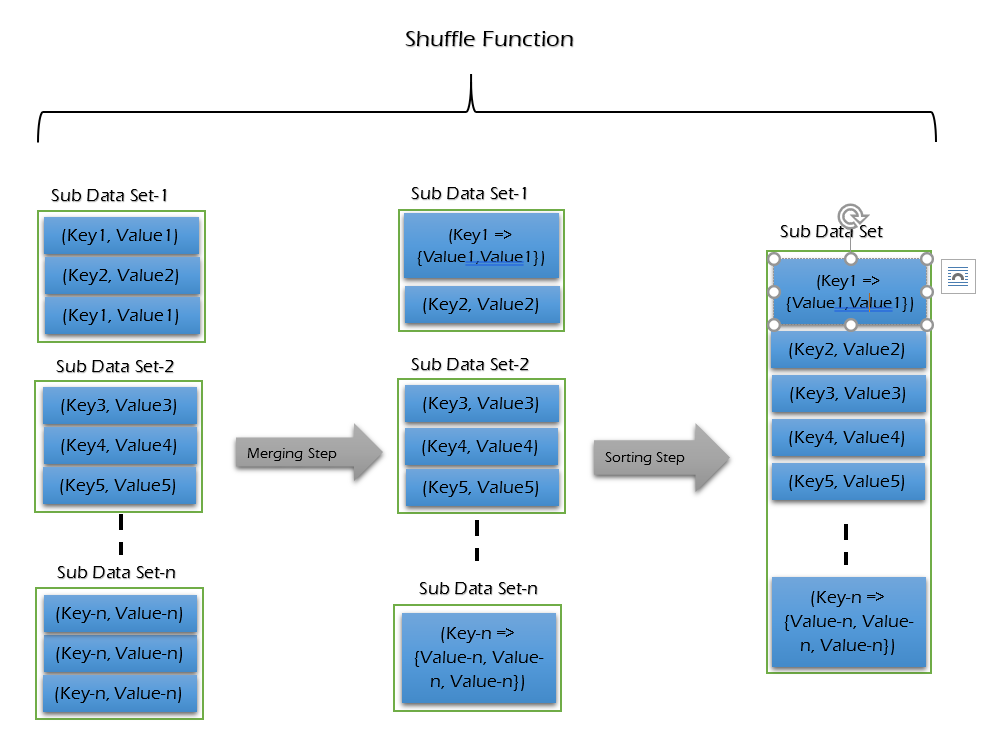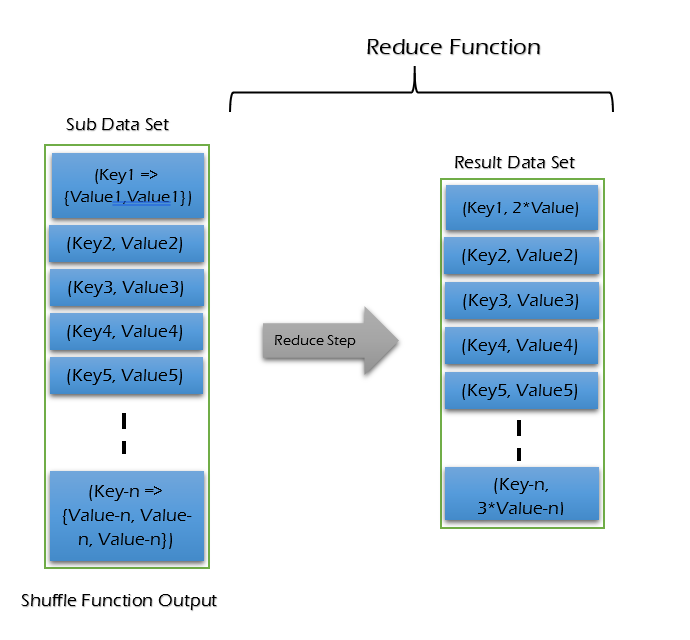###### Summary -

In this topic, we described about the below sections -

• Algorithm

MapReduce is a Distributed Data Processing Algorithm introduced by Google. MapReduce Algorithm is mainly inspired by Functional Programming model. MapReduce algorithm is useful to process huge amount of data in parallel, reliable and efficient way in cluster environments.

It divides input task into smaller and manageable sub-tasks to execute them in-parallel. MapReduce algorithm is based on sending the processing node (local system) to the place where the data exists.

MapReduce Algorithm works by breaking the process into 3 phases.

1. Map Phase
2. Sort & Shuttle phase
3. Reduce phaseIn MapReduce, each phase has key-value pairs as input and output. MapReduce will always expect the input in the form of Key & Value pairs from HDFS layers. Once the MapReduce processing completed, it will produce the o/p again on top of HDFS in the form of (Key, Value) pair.

PhaseInputOutput
Mapper(K,V)(K,V)
Shuffle & Sort(K,V)(K, list(V))
Reducer(K,list(V))(K,V)

## Map Phase -

Map Function is the first step in MapReduce Algorithm. Map phase will work on key & value pairs input. It takes input tasks and divides them into smaller sub-tasks and then perform required computation on each sub-task in parallel.

In the map phase, key & value is in the form of byte offset values. A list of data elements is provided to mapper function called map(). Map() transforms input data to an intermediate output data element.

Mapper output will be displayed in the form of (K,V) pairs. Map phase performs the following two sub-steps -

• Splitting - Takes input dataset from Source and divide into smaller sub-datasets.
• Mapping - Takes the smaller sub-datasets as an input and perform required action or computation on each sub-dataset.

The output of the Map Function is a set of key and value pairs as <Key, Value>.## Shuffle & Sort Phase -

This is the second step in MapReduce Algorithm. Shuffle Function is also known as “Combine Function”. Mapper output will be taken as input to sort & shuffle.

The shuffling is the grouping of the data from various nodes based on the key. This is a logical phase. Sort is used to list the shuffled inputs in sorted order.

It performs the following two sub-steps -

• Merging - combines all key-value pairs which have same keys and returns <Key, List<Value>>.
• Sorting - takes output from Merging step and sort all key-value pairs by using Keys. This step also returns <Key, List<Value>> output but with sorted key-value pairs.

It takes a list of outputs coming from “Map Function” and perform these two sub-steps on each and every key-value pair. Finally, Shuffle Function returns a list of <Key, List<Value>> sorted pairs to Reducer phase.## Reducer Phase -

Reduce phase is the final step in MapReduce Algorithm. Reduce is inherently sequential unless processing multiple tasks. It takes list of <Key, List<Value>> sorted pairs from Shuffle Function and perform reduce operation.

Reduce function receives an iterator values from an output list for the specific key. Reducer combines all these values together and provide single output value for the specific key. This phase performs only one step - Reduce step.

Reduce step <Key, Value> pairs are different from map step <Key, Value> pairs. Reduce step <Key, Value> pairs are computed and sorted pairs. After completion of the Reduce Phase, the cluster collects the data to form an appropriate result and sends it back to the Hadoop server.